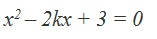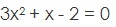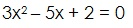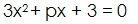Study Materials: CBSE Notes

Our CBSE Notes for Assignment Class 10 maths 4. Quadratic Equations - CBSE Notes - Toppers Study is the best material for English Medium students cbse board and other state boards students.

Notes ⇒ Class 10th ⇒ Mathematics ⇒ 4. Quadratic Equations

# Assignment Class 10 maths 4. Quadratic Equations - CBSE Notes - Toppers Study

Topper Study classes prepares CBSE Notes on practical base problems and comes out with the best result that helps the students and teachers as well as tutors and so many ecademic coaching classes that they need in practical life. Our CBSE Notes for Assignment Class 10 maths 4. Quadratic Equations - CBSE Notes - Toppers Study is the best material for English Medium students cbse board and other state boards students.

## Assignment Class 10 maths 4. Quadratic Equations - CBSE Notes - Toppers Study

CBSE board students who preparing for class 10 ncert solutions maths and Mathematics solved exercise chapter 4. Quadratic Equations available and this helps in upcoming exams 2023-2024.

### You can Find Mathematics solution Class 10 Chapter 4. Quadratic Equations

• All Chapter review quick revision notes for chapter 4. Quadratic Equations Class 10
• NCERT Solutions And Textual questions Answers Class 10 Mathematics
• Extra NCERT Book questions Answers Class 10 Mathematics
• Importatnt key points with additional Assignment and questions bank solved.

NCERT Solutions do not only help you to cover your syllabus but also will give to textual support in exams 2023-2024 to complete exercise maths class 10 chapter 4 in english medium. So revise and practice these all cbse study materials like class 10 maths chapter in english ncert book. Also ensure to repractice all syllabus within time or before board exams for ncert class 10 maths ex in english.

See all solutions for class 10 maths chapter 4 exercise 4 in english medium solved questions with answers.

### Assignment class 10 Mathematics Chapter 4. Quadratic Equations

• Assignment Class 10 Maths 4. Quadratic Equations - CBSE Notes - Toppers Study
• Class 10 Ncert Solutions
• Solution Chapter 4. Quadratic Equations Class 10
• Solutions Class 10
• Chapter 4. Quadratic Equations Assignment Class 10

## Assignment Class 10 maths 4. Quadratic Equations - CBSE Notes - Toppers Study

Questions for practice

1 Marks questions;

Q1.   Write the discriminant of the quadratic equation 3x2 - 5x - 11 = 0.

Q2.   For what value of k quadratic equation x2 - kx + 4 = 0 has equal

roots.

Q3.   Write the nature of the roots of equation 4x2 - 12x + 8 = 0.

Q4.   What will be the value of D when given quadratic equation is a

complete square.

Q5.   Find the value of x in equation x2 - 3 = 0.

Q6.   Write the value of x in equation x2 - 15 = 0.

Q7.   If x = - 2 is a root of the quadratic equation 3x2 - 5x + 2k = 0. Then

find the value of k.

Q8.   For what value of k for which x = 3 is a solution of kx2 - 2x - 1 = 0.

Q9.   For what value of p the quadratic equation 2x2 - 6x + p = 0 has

real and distinct roots.

Q10.  If one root of the equation x2 + 7x + k = 0 is - 2, then find the value

of k and other root.

Q11.  For what value of k the given quadratic equation will be a

complete square.Q12.  Find the solution of quadratic equation ax2 – 2abx = 0.

Q13.  Write a quadratic equation whose two roots are - 3 and 4.

Q14.  Find the nature of equation (x – 2a ) (x – 2b ) = 4ab.

Q15.  What is the middle term of a quadratic equation which roots are

- 4 and - 5 ?

Q16.  2 is the one of the root of quadratic equation 3x2 - 11x + p = 0 then

find the value of p.

Q17.  Write the discriminant of the quadratic equation .Q18.  What is the nature of this equation.Q19.   For what value of k the equation x2 + kx + 4 = 0 has no real roots?

Q20.   For what value of p the equationhas real roots?

Q21.  Find the roots of given quadratic equation by the method of

completing the square.Q22.  Find the value of a nad b such that x = 1, x = - 2 are the solution

of the quadratic equation x2 + ax + b = 0.

Q23.   Solve for x

abx2 + (b2 - ac) x - bc = 0

Q24.   solve for x

3y2 + (6 + 4a)y + 8a = 0

Q25.  Divide 51 in to two parts such that their product is 378.

Q26.  if the roots of the equation (b-c)x2 + (c - a)x + (a - b) = 0 are equal

then prove that 2b = a + c.

Q27.  Find the value of k from the given equation so that equation

has equal roots.Q28.  Product of two number is 36 and difference is 9, find those number.

Q29.  Find the value of k if the equation has two equal and real roots.

Q30. Find the nature of following quadratic equations:

(A)  x2 + 4x + 9 = 0

(B)  3x2 - 2x - 8 = 0

(C)  11x2 + 8x - 3 = 0

(D)  x2 + 7x + 4 = 0

(E)  x2 + 4x + 4 = 0

(F)  3x2 + 10x + 8 = 0

(G) 4x2 + 3x + 5 = 0

(H)  7x2 + 12x + 5 = 0

(I)  5x2 - 10x + 5 = 0

Q31. A journey of 192km from Mumbai to Pune takes 2hrs less by a fast train then by a slower train. If the speed of the slower train is 16 km/hr less than the faster train, find the speed of each train.

Q32. A part of monthly expenses of a family is constant and the remaining varies with the price of wheat. When the rate of wheat is Rs.250 per quintal, the total monthly expense if the family is Rs.1000 and when it is Rs.240 per quintal, the total monthly expense is Rs.980. Find the total monthly expenses of the family when cost wheat is Rs.350 per quintal.

Q33. A man travels 600km partly by train and partly by car. If he covers 400km by train and rest by car, it takes him 6hrs and 30minutes. But if he travels 200km by train and rest by car, he takes half an hour more. Find the speed of the train and that of the car.

##### Other Pages of this Chapter: 4. Quadratic Equations

Important Study materials for classes 06, 07, 08,09,10, 11 and 12. Like CBSE Notes, Notes for Science, Notes for maths, Notes for Social Science, Notes for Accountancy, Notes for Economics, Notes for political Science, Noes for History, Notes For Bussiness Study, Physical Educations, Sample Papers, Test Papers, Mock Test Papers, Support Materials and Books.Mathematics Class - 11th

NCERT Maths book for CBSE Students.

books

## Study Materials List:

##### Notes ⇒ Class 10th ⇒ Mathematics
1. Real Numbers
2. Polynomials
3. Pair of Linear Equations in Two Variables
5. Arithmetic Progressions
6. Triangles
7. Coordinate Geometry
8. Introduction to Trigonometry
9. Some Applications of Trigonometry
10. Circles
11. Constructions
12. Areas Related to Circles
13. Surface Areas and Volumes
14. Statistics
15. Probability

New Books